# Methane (CH4) lewis dot structure, molecular geometry, electron geometry, polar or nonpolar, bond angle

Home  > Chemistry Article > CH4 lewis structure and its molecular geometryMethane is a colorless and odorless gas formed from one atom of carbon and four atoms of hydrogen having the chemical formula CH4. It is the simplest of saturated hydrocarbons. It is used for the generation of electricity by burning as a fuel in steam generator or gas turbine.

In this article, we will discuss Methane (CH4) lewis dot structure, molecular geometry, electron geometry, hybridization, polar or nonpolar, its bond angle, etc.

Methane is mainly used as a fuel for turbines, water heaters, ovens, etc. It is also used in industrial chemical processes to prepare other chemicals.

Properties of Methane

• It has a molar mass of 16.043 g/mol.
• It has a boiling point of −161.5 °C and a melting point of −182.456 °C.
• It is soluble in ethanol, ether, benzene, etc but insoluble in water.
• It is a nontoxic and flammable gas.
• Methane is easily ignited.
 Name of Molecule Methane Chemical formula CH4 Molecular geometry/shape of CH4 Tetrahedral Electron geometry of CH4 Tetrahedral Hybridization Sp³ Bond angle 109.5° Total Valence electron for CH4 8 The formal charge of CH4 0
Page Contents

## How to draw lewis structure of CH4 (Methane)?

CH4 lewis’s structure is very simple and easy to draw, it is made up of one carbon atom that takes the central position and four hydrogens that spread around the central atom. There are no lone pair electrons present in the lewis dot structure of CH4.

Let’s see how to draw the lewis structure of CH4 by following some simple steps-

## Simple steps for drawing the Lewis dot structure for CH4

1. Count total valence electron in CH4

In the first step, we have to find how many valence electrons are there in CH4, so that we can distribute them around central and terminal atoms with the goal of completing their octet shell.

You have two ways to find out the valence electron for a particular atom, either by looking at their periodic group or by writing their electronic configuration. We will use the method of the periodic group for finding the valence electron in CH4.

The carbon atom belongs to Group 4A or 14A in the periodic table, hence, it has a 4 valence electron in its outermost shell whereas the hydrogen atom belongs to Group 1A, hence, it has only 1 valence electron in its outermost shell.

⇒ Total valence electron in Carbon = 4

⇒ Total valence electron in Hydrogen = 1

∴ Total valence electron available for drawing the CH4 lewis structure = 4 + 1*4 = 8 valence electrons     [∴CH4 has four hydrogen atoms and one carbon atom]2. Find the least electronegative atom and placed it at center

Whenever hydrogen is present in any molecule then it doesn’t matter which atom is less or more electronegative, hydrogen always goes outside in a lewis diagram and it needs only two electrons to complete its outer shell.

So, place all Hydrogen atoms outside in the lewis diagram and the Carbon atom at the central position.3. Connect each outer atom to the central atom with a single bond

In this step, we will simply connect each outer atom (which is hydrogen in the CH4 molecule) to the central atom(carbon) with the help of a single bond.Now look at this structure and count how many electrons we used till now and how many are left.

As four single bonds are used in the above structure that contains 8 electrons(1 single bond means 2 electrons) and we have a total of 8 valence electrons are available for drawing the CH4 lewis structure.

∴ (8 – 8) = 0 valence electrons

So, we used all available valence electrons in the above structure, now just count the electrons in the valence or outer shell of each atom to know if they completed their octet or not.

“The octet rule refers to the tendency of atoms to prefer to have eight electrons in the valence shell”.

The atom which have less or more than 8 electrons in valence shell, they are classified as Exceptional to the octet rule.

The carbon atom needs 8 electrons in its valence shell to complete the octet, if you see the above structure, the carbon central atom is attached with four single bonds, which means, it has already 8 electrons in its outer shell, hence, the carbon atom completed its octet comfortably.

Note: The hydrogen atom only requires two valence electrons for completing the octet as it is exceptional to the octet.

So, in the above structure, each hydrogen atom is attached to the single bond, which means, they already have two electrons in their outer shell, hence, hydrogen atoms also completed their octet rule comfortably.### CH4 lewis structure

As you see in this CH4 lewis structure, carbon central atom and hydrogen atom completed their octet, and everything looks fine, but, for the sake of satisfaction, we should also determine the formal charge in the above structure to know whether it is stable or not.

4. Check the stability with the help of a formal charge concept

A formal charge is the charge assigned to an atom in a molecule, assuming that electrons in all chemical bonds are shared equally between atoms.

The structure with the formal charge close to zero or zero is the best and stable lewis structure.

To calculate the formal charge in the CH4 lewis structure. Use the formula given below-Let’s start with the central atom which is carbon in the CH4 molecule.

For carbon atom:

⇒ Valence electrons of carbon = 4

⇒ Lone pair electrons on carbon = 0

⇒ Bonding electrons of carbon(4 single bonds) = 8

∴ (4 – 0 – 8/2) = 0 formal charge on the carbon central atom.

Each hydrogen atom in the CH4 lewis structure has the same number of bonded pair electrons, hence, just count formal charge for just one hydrogen atom, rest all will follow it.

For hydrogen atom

⇒ Valence electrons of hydrogen = 1

⇒ Lone pair electrons on hydrogen = 0

⇒ Bonding electrons around hydrogen (1 single bond) = 2

∴ (1 – 0 – 2/2) = o formal charge on each hydrogen atom.

Hence, each atom in CH4 gets a formal charge equal to zero.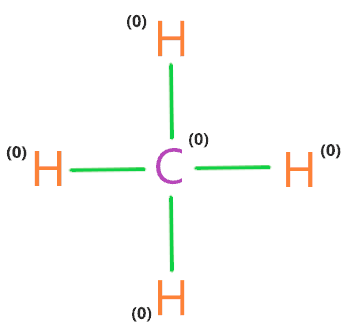Therefore, the above lewis structure of CH4 (Methane) is the most stable, appropriate, and reliable.

## What are the electron and molecular geometry of CH4 (Methane)?

The molecular geometry of CH4 is tetrahedral as the carbon central atom has no lone pair and is attached to the four hydrogen atoms with the help of a single covalent bond. So, there are four regions of electron density around the carbon central atom”.

The electron pair around the carbon central atom will repel each other and tried to go far from each other, they will take the position where repulsion becomes minimum between them.

According to the VSEPR theory, “in most of the cases, the central atom with four regions of density adopt a tetrahedral structure because repulsion is minimum in electron pairs at this position.”In the above figure, the red lines outline the tetrahedron and the black line shows the bonded pair or electron pair formed between carbon and hydrogen. “The carbon atom is located in the center of the tetrahedron, while the four hydrogens atoms are located on the vertices.”

So, there are four bonding pairs formed in CH4 molecule, hence, according to the VSEPR theory, they will repel each other, as a result, all corners atoms(hydrogens) spread out as much as they can and takes the place where the repulsion is minimum and stability is much better.

Hence, the final molecular geometry of CH4 appears like a regular tetrahedron with a bond angle ∠HC−H =109.5°.

If you can’t visualize the molecular geometry of CH4, then theoretically we can use an AXN method and VSEPR chart to determines its shape.

AXN is a simple formula that represents the number of the bonded atom and lone pair on the central atom to predict the shape of the molecule using the VSEPR chart.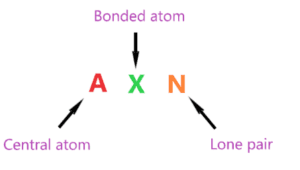AXN notation for CH4 molecule:

• A denotes the central atom, so, in the CH4 molecule, carbon is the central atom. A = Carbon
• X denotes the bonded atoms to the central atom, as we know, the carbon central atom is bonded with four hydrogen atoms. Therefore, X = 4
• N denotes the lone pair on the central atom, as per CH4 lewis structure, carbon central atom doesn’t contain any lone pair. Hence, N = 0

So, the AXN notation for the CH4 molecule becomes AX4N0 or AX4.

As per the VSEPR chart, if a molecule’s central atom is attached with four bonded atoms and has contained zero lone pair on the central atom then the molecular geometry of that molecule is tetrahedral in nature, and its electron geometry is also tetrahedral.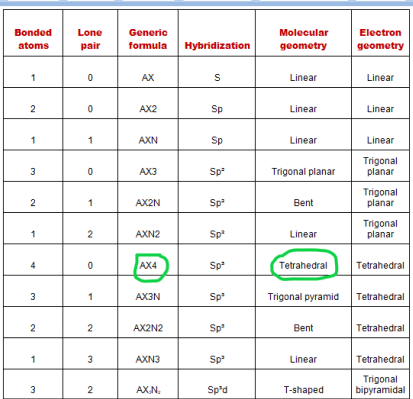Hence, the molecular geometry for CH4 is tetrahedral and its electron geometry is also tetrahedral.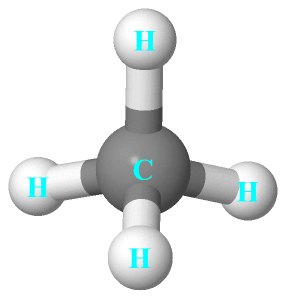## Hybridization of CH4

Let’s find the hybridization of CH4 through the steric number of its central atom.

“Steric number is the addition of a total number of bonded atoms around a central atom and the lone pair present on it.”

∴ Steric number of CH4 = (Number of bonded atoms attached to carbon atom + Lone pair on carbon atom)

As per the lewis structure of CH4, the central Carbon atom is bonded with four hydrogen atoms and it doesn’t have any lone pair.

∴ Steric number of CH4 = (4 + 0) = 4

 Steric number Hybridization 1 S 2 Sp 3 Sp² 4 Sp³ 5 Sp³d 6 Sp³d²

So, for a steric number of four, we get the Sp3 hybridization on a carbon atom in the CH4 molecule.

The newly produced hybrid orbitals in CH4 molecule have 25% behavior of the s orbital and 75% behavior of the p orbital.

## The bond angle of CH4

As we know the molecular geometry of CH4 is regular tetrahedral with no distortion, hence, according to the VSEPR theory, for a regular tetrahedral structure, the bonded atoms around the central atom will spread at an angle of approx 109.5° to minimize the repulsion and attains stability.

Hence, the bond angle of ∠HC−H in CH4 is 109.5°.## Methane polarity: is CH4 polar or nonpolar

Well, we know the polar molecule has some dipole moment because of unequal distribution of charges whereas the non-polar molecule has an equal distribution of charges that cause zero dipole moment because they cancel out each other due to the symmetrical shape of the molecule.

Is Methane (CH4) polar or non-polar? CH4 is a non-polar molecule because it contains four bonds(C-H) that are arranged symmetrically in tetrahedral geometrical shapes. Due to this, dipole moment generated on each side along C-H will cancel out each other making an overall nonpolar molecule.

Let’s understand whether CH4 is polar or non-polar in detail.

Three factors that indicate the polarity of CH4

1. Electronegativity:

It directly influences the polarity nature of any atom or molecule because the polarity is directly proportional to the difference of electronegativity created by atoms or molecules. Electronegativity means the tendency of an atom to attracting electrons towards itself.

If the electronegativity difference between the atoms is high then the polarity will also be higher. Now, look at the electronegativity of carbon and hydrogen.

As carbon electronegativity is around 2.6 and for hydrogen, it is around 2.2. Therefore, carbon has a higher tendency to attract an electron to itself than hydrogen.

Also, the electronegativity difference between carbon and hydrogen is less than 0.5, and according to the Pauling scale if the electronegativity difference between atoms is lower than 0.5 then the bond between that atoms behaves as nonpolar.

Note: It doesn’t necessary if some bond is polar then the whole molecule can also be polar. In the case of CO2, the C-O bond is polar in nature due to the difference in electronegativity between them is more than 0.5. But the whole molecule of CO2 is non-polar in nature due to its symmetrical shape.

Therefore, if we consider the C-H bonds polar, then all dipoles generated along these bonds will cancel out each other because of the symmetrical tetrahedral geometry of CH4 resulting in an overall nonpolar molecule.

2. Dipole moment

Dipole moment ensures the strength of polarity between carbon and hydrogen atoms. As greater the dipole moment of the molecule, the more is the polar nature of that molecule. The electronegativity difference between the atoms(Carbon and hydrogen) induced positive and negative charges.

As carbon is more electronegative than hydrogen, hence, some negative charge is induced on the carbon atom and a partial positive charge is induced on the hydrogen atoms. So, these charges create four dipole moments along with C–H bond. Let’s see the figure given below.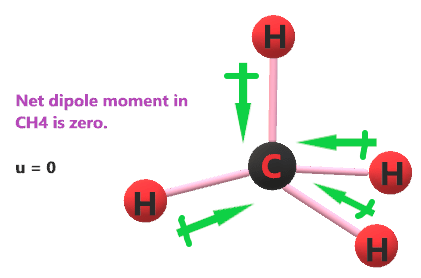As dipole moment induced in the direction from hydrogen to carbon because carbon is more electronegative than hydrogen, so, it attracts electrons towards itself. But dipole moments generated along these bonds can easily be canceled out of each other because the CH4 has a regular structure of tetrahedral with no lone pair on the central atom.

In mathematical terms, we can express dipole moment as D = Q×R

Dipole moment formula = charge on the atoms * the distance between them.

3. Geometrical or molecular shape:

The geometrical structure of any molecule has great influences on the polarity nature. As the CH4 molecular geometry is tetrahedral that has four nonpolar covalent bonds (C-H) but all they are in the opposite direction. Also, a central atom of CH4 doesn’t contain any lone pair which makes its molecule shape highly symmetric.

So, the C-H bonds are highly symmetric and opposite in direction which can easily be canceled out each other causing this molecule to become non-polar in nature.

## FAQ

### Why is the molecular geometry of CH4 is same as its electron geometry?

The molecular geometry of CH4 is tetrahedral and its electron geometry is also tetrahedral because as per VSEPR theory, molecular shape considers only bond pairs or atoms while electron geometry considers bonded atoms as well as lone pairs present on the central atom.

According to the lewis structure of CH4, the central atom(carbon) doesn’t contain any lone pair on it.

Hence, only bonded atoms are used to determine the geometry of CH4.

Therefore, Molecular geometry of CH4 = Electron geometry of CH4     [∴ no lone pair on central atom of CH4]

### How many lone pairs and bond pairs are present around the carbon central atom in the CH4 lewis structure?

According to the lewis dot structure of CH4, the Carbon central atom doesn’t contain any lone pair but is attached to the four hydrogen atoms with the help of four bonded pairs.

## Summary

• The total valence electron available for drawing the Methane (CH4) lewis structure is 8.
• The steric number of the central atoms in methane is 4 that ensures that it has an Sp3 hybridization.
• CH4 is a nonpolar molecule due to its symmetrical geometry that causes uniform charge distribution all over the atom leads to a zero net dipole moment and makes this molecule non-polar in nature.
• The molecular geometry or shape for CH4 is the tetrahedral with bond angle ∠HC−H =109.5°.
• The electron geometry for CH4 is also tetrahedral as it central has 4 regions of electron density with no lone pair on it.
• Lewis dot structure of CH4 contains only 4 bonded pairs(8 shared electrons) and doesn’t contain any lone pair electrons in the molecule.
##### Subscribe to Blog via Email

Join 2 other subscribers

Share it...